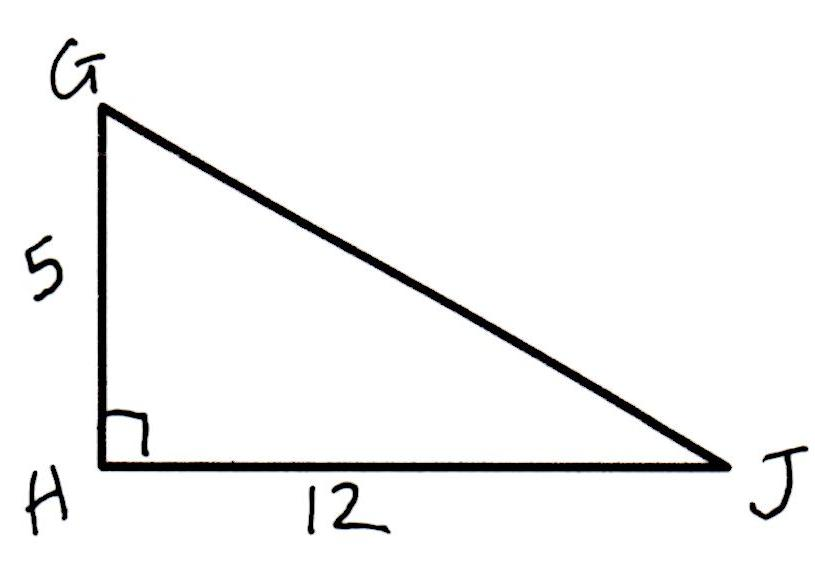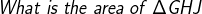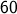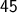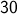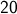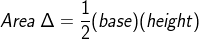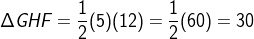# Basic Geometry : How to find the area of a right triangle

## Example Questions

← Previous 1 3 4 5 6 7 8 9

### Example Question #1 : How To Find The Area Of A Right Triangle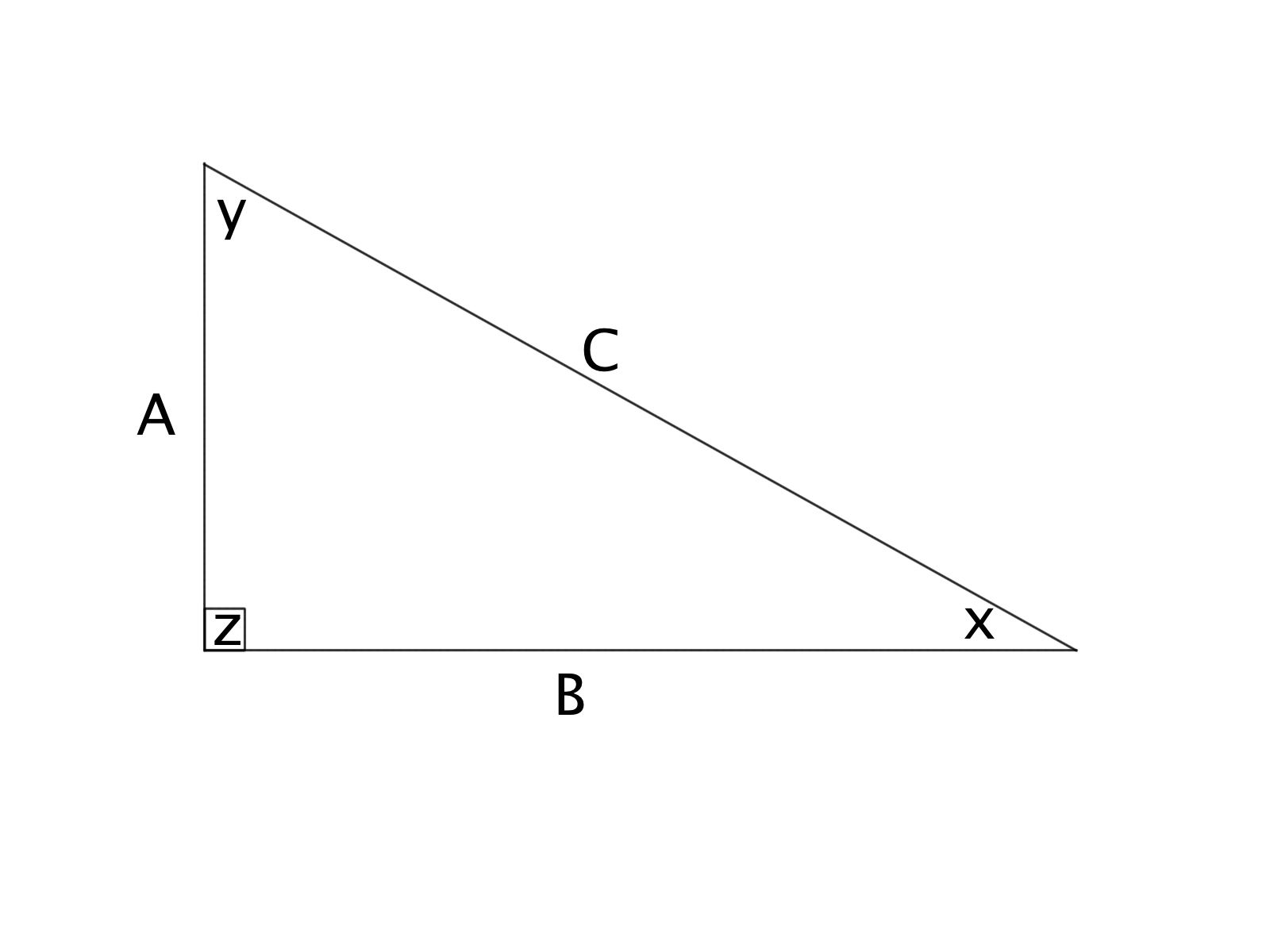Given:

A = 3 cm

B = 4 cm

What is the area of the right triangle ABC?

6 square centimeters

5 square centimeters

12 square centimeters

7 square centimeters

13 square centimeters

6 square centimeters

Explanation:

The area of a triangle is given by the equation: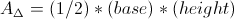Since the base leg of the given triangle is 4 cm, while the height is 3 cm, this gives: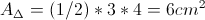### Example Question #2 : How To Find The Area Of A Right TriangleGiven:

A = 4 cm

B = 6 cm

What is the area of the right triangle ABC?

24 square centimeters

10 square centimeters

11 square centimeters

12 square centimeters

8 square centimeters

12 square centimeters

Explanation:

The area of a triangle is given by the equation:Since the base leg of the given triangle is 4 cm, while the height is 3 cm, this gives: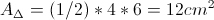### Example Question #3 : How To Find The Area Of A Right TriangleGiven:

A = 3 cm

B = 7 cm

What is the area of the triangle?

8.3 square centimeters

10 square centimeters

10.5 square centimeters

7 square centimeters

7.6 square centimeters

10.5 square centimeters

Explanation:

The area of a triangle is given by the equation:Since the base leg of the given triangle is 4 cm, while the height is 3 cm, this gives: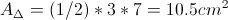### Example Question #4 : How To Find The Area Of A Right TriangleGiven that:

A = 6 cm

B = 10 cm

What is the area of the right trianlge ABC?

16 square centimeters

60 square centimeters

30 square centimeters

90 square centimeters

35 square centimeters

30 square centimeters

Explanation:

The area of a triangle is given by the equation:Since the base leg of the given triangle is 4 cm, while the height is 3 cm, this gives: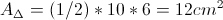### Example Question #5 : How To Find The Area Of A Right TriangleGiven that:

A = 3 cm

B = 4 cm

C = 5 cm

What is the area of the right triangle ABC?

7 square centimeters

10 square centimeters

12 square centimeters

6 square centimeters

6.5 square centimeters

6 square centimeters

Explanation:

The area of a triangle is given by the equation:Since the base leg of the given triangle is 4 cm, while the height is 3 cm, this gives: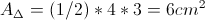### Example Question #6 : How To Find The Area Of A Right TriangleGiven that:

A = 10 cm

B = 20 cm

What is the area of the right triangle ABC?

70 square centimeters

50 square centimeters

120 square centimeters

100 square centimeters

30 square centimeters

100 square centimeters

Explanation:

The area of a triangle is given by the equation:Since the base leg of the given triangle is 4 cm, while the height is 3 cm, this gives: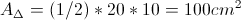### Example Question #7 : How To Find The Area Of A Right Triangle

The length of the legs of the triangle below (not to scale) are as follows: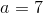cm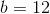cm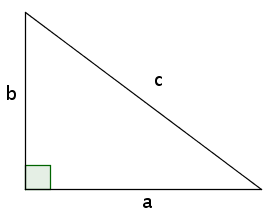What is the area of the triangle?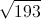square centimeters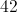linear centimeterssquare centimeters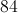square centimeters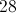square centimeterssquare centimeters

Explanation:

The formula for the area of a triangle is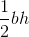whereis the base of the triangle andis the height.

For the triangle shown, sideis the base and sideis the height.

Therefore, the area is equal to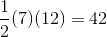or, based on the units given, 42 square centimeters

### Example Question #8 : How To Find The Area Of A Right Triangle

An equilateral triangle has a side of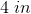What is the area of the triangle?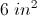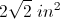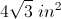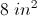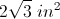Explanation:

An equilateral triangle has three congruent sides. The area of a triangle is given by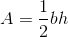whereis the base andis the height.

The equilateral triangle can be broken into two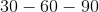right triangles, where the legs areandand the hypotenuses is.

Using the Pythagorean Theorem we getor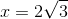and the area is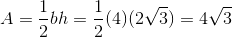### Example Question #9 : How To Find The Area Of A Right Triangle

The hypotenuse of a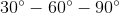triangle measures eight inches. What is the area of this triangle (radical form, if applicable)?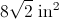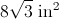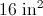It is impossible to tell from the information given.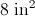Explanation:

In a, the shorter leg is half as long as the hypotenuse, and the longer leg is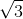times the length of the shorter. Since the hypotenuse is 8, the shorter leg is 4, and the longer leg is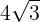, making the area: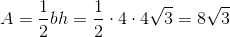### Example Question #10 : How To Find The Area Of A Right Triangle